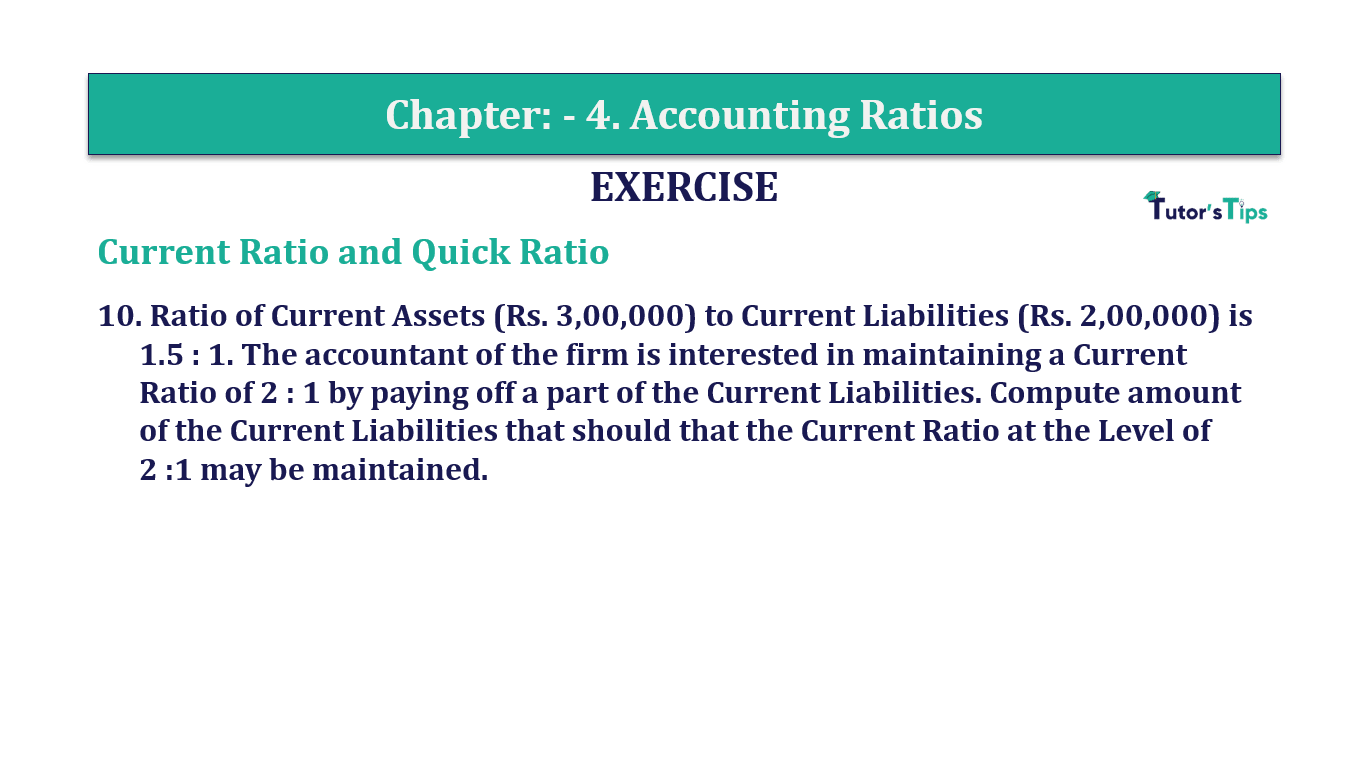# Question 10 Chapter 4 of +2-B – T.S. Grewal 12 ClassQuestion No. 10 - Chapter No.4 - T.S. Grewal +2 Book Part B

Question 10 Chapter 4 of +2-B

Current Ratio and Quick Ratio

10. Ratio of Current Assets (Rs. 3,00,000) to Current Liabilities (Rs. 2,00,000) is 1.5 : 1. The accountant of the firm is interested in maintaining a Current
The ratio of 2: 1 by paying off a part of the Current Liabilities. Compute the amount of the Current Liabilities that should that the Current Ratio at the Level of  2:1 may be maintained.

### The solution of Question 10 Chapter 4 of +2-B: –

 Current Ratio = Current Assets = 1.5 Current Liabilities 1

Current Liabilities=Rs. 1,75,000

The company is interested in maintaining Current Ratio 2: 1 by paying off the liability.

∴ Let the liability paid-off by the company = x

 New Current Assets = 3,00,000 – x = 2 2,00,000 – x 1

or,Rs. 4,00,000 – 2x =Rs. 3,00,000 – x
x=Rs. 1,00,000
Therefore, the liability of Rs. 1,00,000 need to be paid off by the company in order to maintain the Current Ratio of 2: 1.

Balance Sheet: Meaning, Format & Examples

Comment if you have any question.

Also, Check out the solved question of previous Chapters: –